BUOY

## Turbine Drag

The theoretical maximum power output and drag coefficient for the CoRMaT turbine is derived by assuming that the rotors are replaced by a disc, which withdraws energy from the water passing through it. At a certain distance downstream of this disc, the water that has passed through flows with a reduced velocity. The theoretical maximum power output and drag coefficient are derived as follows: (Betz, 1966)
Velocity upstream of turbine: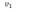Velocity at turbine rotors: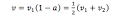where a is the axial induction factor. Velocity downstream of turbine: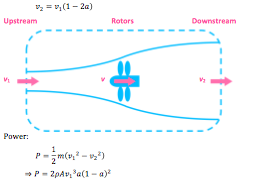where m is the mass of water, A is the area of the rotor and rho is the density of water.
At maximum power: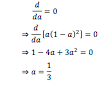Force in axial direction: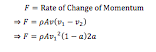Force at maximum power: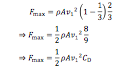where CD is the drag coefficient.